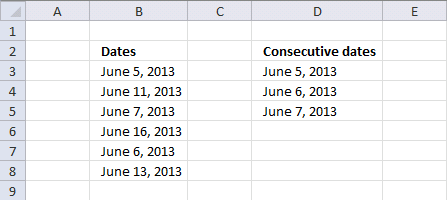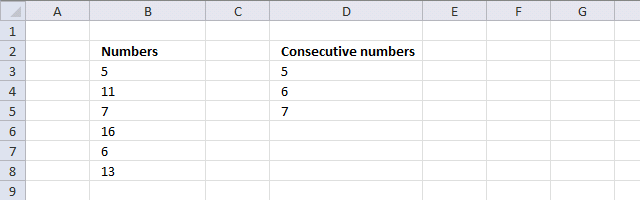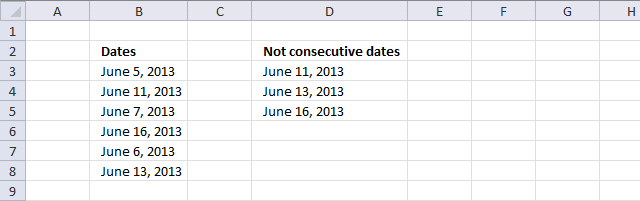Author: Oscar Cronquist Article last updated on June 07, 2013

Column B contains random dates. The array formula in column D returns consecutive dates from column B.Array formula in cell D3:

=IFERROR(SMALL(IF((COUNTIF(\$B\$3:\$B\$8, \$B\$3:\$B\$8+1) + COUNTIF(\$B\$3:\$B\$8, \$B\$3:\$B\$8-1))>0, \$B\$3:\$B\$8, ""),ROW(A1)), "")

How to enter an array formula

1. Select cell D3
2. Copy array formula (Ctrl + c)
3. Click in formula bar
4. Paste formula (Ctrl  + v)
5. Press and hold CTRL + SHIFT
6. Press Enter simultaneously
7. Release all keys

How to copy array formula

1. Select cell D3
2. Copy (Ctrl + c)
3. Select cell range D4:D5
4. Paste (Ctrl + v)

### Find consecutive numbers

Since dates are numbers in excel you can also use the array formula to identify consecutive numbers.### Find non-consecutive datesArray formula in cell D3:

=IFERROR(SMALL(IF((COUNTIF(\$B\$3:\$B\$8, \$B\$3:\$B\$8+1)+COUNTIF(\$B\$3:\$B\$8, \$B\$3:\$B\$8-1))=0, \$B\$3:\$B\$8, ""), ROW(A1)), "")

### Explaining the array formula in cell D3 (Consecutive dates)

Step 1 - Find dates that have a consecutive date except the last consecutive date

COUNTIF(\$B\$3:\$B\$8,\$B\$3:\$B\$8+1)

becomes

=COUNTIF({41430; 41436; 41432; 41441; 41431; 41438}, {41430; 41436; 41432; 41441; 41431; 41438}+1)

becomes

=COUNTIF({41430; 41436; 41432; 41441; 41431; 41438}, {41431; 41437; 41433; 41442; 41432; 41439})

and returns

{1;0;0;0;1;0}

If you are interested in how the COUNTIF function works, read this post: COUNTIF function

Step 2 - Find dates that have a consecutive date except the first consecutive date

COUNTIF(\$B\$3:\$B\$8,\$B\$3:\$B\$8-1)

becomes

=COUNTIF({41430; 41436; 41432; 41441; 41431; 41438}, {41430; 41436; 41432; 41441; 41431; 41438}-1)

becomes

=COUNTIF({41430; 41436; 41432; 41441; 41431; 41438}, {41429; 41435; 41431; 41440; 41430; 41437})

and returns

{0;0;1;0;1;0}

Step 3 - Add arrays using OR logic

COUNTIF(\$B\$3:\$B\$8,\$B\$3:\$B\$8+1)+COUNTIF(\$B\$3:\$B\$8,\$B\$3:\$B\$8-1)

becomes

{1;0;0;0;1;0} + {0;0;1;0;1;0} and returns {1;0;1;0;2;0}

Step 4  - Find values in array larger than 0 (zero)

(COUNTIF(\$B\$3:\$B\$8,\$B\$3:\$B\$8+1)+COUNTIF(\$B\$3:\$B\$8,\$B\$3:\$B\$8-1))>0

becomes

{1;0;1;0;2;0}>0

and returns {TRUE; FALSE; TRUE; FALSE; TRUE; FALSE}

Step 5 - Replace TRUE with corresponding date and FALSE with blanks

IF((COUNTIF(\$B\$3:\$B\$8, \$B\$3:\$B\$8+1) + COUNTIF(\$B\$3:\$B\$8, \$B\$3:\$B\$8-1))>0, \$B\$3:\$B\$8, "")

becomes

IF({TRUE; FALSE; TRUE; FALSE; TRUE; FALSE}, \$B\$3:\$B\$8, "")

becomes

IF({TRUE; FALSE; TRUE; FALSE; TRUE; FALSE}, {41430; 41436; 41432; 41441; 41431; 41438}, "")

and returns {41430; ""; 41432; ""; 41431; ""}

Interested in how the IF function works, read this post: IF function explained

Step 6 - Find the n-th smallest date

SMALL(IF((COUNTIF(\$B\$3:\$B\$8, \$B\$3:\$B\$8+1) + COUNTIF(\$B\$3:\$B\$8, \$B\$3:\$B\$8-1))>0, \$B\$3:\$B\$8, ""),ROW(A1))

becomes

SMALL({41430; ""; 41432; ""; 41431; ""}, ROW(A1))

becomes

SMALL({41430; ""; 41432; ""; 41431; ""}, 1)

and returns 41430 (June 5, 2013) in cell D3.

Interested in how the SMALL function works, read this post:
SMALL function and LARGE function

The ROW function: ROW function explained

### Conditional formatting consecutive datesConditional formatting formula:

=(COUNTIF(\$B\$3:\$B\$8,B3+1)+COUNTIF(\$B\$3:\$B\$8,B3-1))>0

How to apply conditional formatting

1. Select cell range B3:B8
2. Go to "Home" tab
3. Click "Conditional formatting" button
4. Click "New Rule..."
5. Click "Use a formula to determine which cells to format"
6. Format values where this formula is TRUE:
=(COUNTIF(\$B\$3:\$B\$8,B3+1)+COUNTIF(\$B\$3:\$B\$8,B3-1))>0
7. Click "Format.." button
8. Go to "Fill" tab
9. Pick a color
10. Click OK
11. Click OK

Consecutive dates.xlsx

### Functions in this post

COUNTIF(rangecriteria)
Counts the number of cells within a range that meet a single criterion that you specify.

IF(logical_test, [value_if_true], [value_if_false])
Checks whether a condition is met, and returns one value if TRUE, and another value if FALSE

SMALL(arrayk)
Returns the k-th smallest value in a data set

ROW(reference)
Returns the row number of a reference.